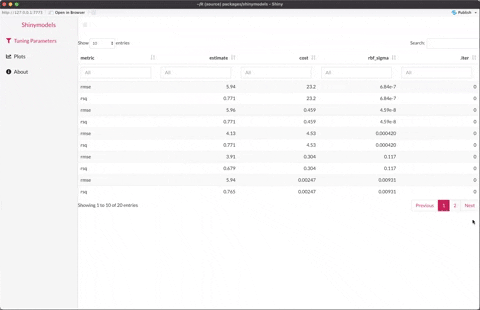`Tidymodels` is the group of packages that allows modeling in R with a tidyverse-friendly interface. It is a commonly used package ecosystem for modeling in R, to learn more about using '{tidymodels}' for modeling, click here. In any kind of modeling, resampling methods, such as cross-validation or validation sets tuning parameters, and model tuning tools are widely used to evaluate the modeling process and select effective models. Once models are created using resampling function like `tune::fit_resamples()` and tuning functions like `tune::tune_grid()` and `tune::tune_bayes()`, the goal is to measure the performance and variation in model results. To make this exploration of a `{tidymodels}` object easier and faster, we built `{shinymodels}`.

##### {shiny} + {tidymodels} = {shinymodels}

As the name suggests, `{shinymodels}` is the package that allows exploring a {tidymodels} object in a shiny application. The goal is to visualize the results of resampling and tuning functions and compare the different models. Through various diagnostic plots, we hope to help identify structures and problematic observations that could otherwise go missing. Also, linking multiple graphics can lead to insight not revealed by a single view. With a model with tuning parameters, users can compare the models for different selections of the parameters.

Let's look at a simple example of how to use the package. Below is the code modeling `mtcars` with tuning parameters using `tune_bayes()` function.

``````    ```{r}
library(tidymodels)
tidymodels_prefer()

set.seed(6735)

folds <- vfold_cv(mtcars, v = 5)
car_rec <-
recipe(mpg ~ ., data = mtcars) %>%
step_normalize(all_predictors())
svm_mod <-
svm_rbf(cost = tune(), rbf_sigma = tune()) %>%
set_engine("kernlab") %>%
set_mode("regression")
# Use a space-filling design with 7 points
set.seed(3254)
svm_res <-
tune_bayes(
svm_mod,
car_rec,
resamples = folds,
initial = 7,
iter = 3,
control = control_bayes(save_pred = TRUE, verbose = TRUE)
)
```
``````

Here, the object svm_res is the `{tidymodels}` object of class `tune_results`. Now, you can use `{shinymodels}` to explore the object in a shiny app. The launcher function in `{shinymodels}` is `explore()`.

``````    ```{r}
library(shinymodels)
explore(svm_res)
```
``````

It should launch the following shiny app:

###As you can see, there are mainly two categories of tabs in the app:

• Tuning Parameters tab: For models with tuning parameters (e.g., `tune_bayes()`), the tab with tuning parameters consists of the data table for the tuning parameters and performance metrics for each candidate model. When the app is first launched, the default configuration is based on the optimal value of the first metric. Selecting a row in the tuning parameter table selects the related model which should then update the diagnostic plots in the plots tab(s).

• Plots tab (s): The Plots tab consists of diagnostic plots to explore the model object. Clicking on a particular point or selecting a group of points in any plot should highlight the point(s) in all other plots. This will help see where an observation lies in different diagnostic plots and get insights about the observation. This will help diagnose the outliers or "problematic" observations in the modeling object and hopefully give insights on why that is the case. In the case of a model with tuning parameters, the plots tab displays the diagnostic plots for the selected model.

We hope that `{shinymodels}` will make it easy, fun, and efficient to explore a `{tidymodels}` object. Especially for users new to both the packages, we hope this package will remove the initial barrier to learn shiny applications in R to explore their modeling object in R.

This package was built by Shisham Adhikari (UC Davis) under the supervision of Max Kuhn (RStudio) and Julia Silge (RStudio) as a part of the RStudio summer internship 2021. Your suggestions, feedback, complaints, or compliments are highly valued and will guide us to improve the package continuously. Please email them to shishamadhikari6@gmail.com or max@rstudio.com or julia.silge@rstudio.com.

To give feedback, file a bug report, or ask a question, go to the GitHub issues page or go to RStudio Community and create a post with the tag `tidymodels`.